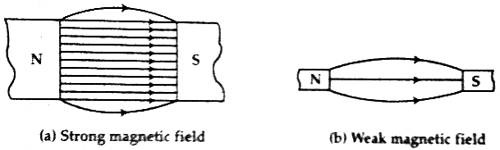# Magnetic Flux

Magnetic flux: Magnetic field is generally represented by magnetic field lines. If the magnetic field is strong then the field lines are closer and densely populated [Fig. (a)]; on the other hand, if the magnetic field is weak, then field lines are thinner i.e., gaps between the lines are wider [Fig. (b)]. In figure strong and weak field lines have been shown between the two poles of the horseshoe magnet. The number of field lines depends on the magnitude of the magnetic field.[N. B. It should be remembered that field lines are totally fictitious, they do not have any existence in nature.]

Now if a plane (real or fictitious) is taken in the magnetic field, then the field lines passing through that place are called magnetic flux (figure-2).Definition of magnetic flux: Number of field lines passing through either a real or a fictitious plane in a magnetic field is called magnetic flux. It is a measurement of the total magnetic field which passes through a given area. It is a useful tool for helping describe the effects of the magnetic force on something occupying a given area.

It is denoted by φ.

Unit: The S. I. unit of magnetic flux is Weber (Wb) or NmA-1.

1 weber: Number of flux that changes per second in a coil for the production of 1-volt potential difference in that coil is called 1 Weber.

In the case of an electric generator where the magnetic field penetrates a rotating coil, the area used in defining the flux is the projection of the coil area onto the plane perpendicular to the magnetic field.